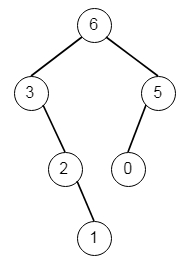# Maximum Binary Tree in C++

C++Server Side ProgrammingProgramming

#### C in Depth: The Complete C Programming Guide for Beginners

45 Lectures 4.5 hours

#### Practical C++: Learn C++ Basics Step by Step

Most Popular

50 Lectures 4.5 hours

#### Master C and Embedded C Programming- Learn as you go

66 Lectures 5.5 hours

Suppose we have an integer array. All elements in that array is unique. A maximum tree building on this array is defined as follow −

• The root will hold the maximum number in the array.

• The left subtree is the maximum tree constructed from left side of the subarray divided by the maximum number.

• The right subtree is the maximum tree constructed from right side of subarray divided by the maximum number.

We have to construct the maximum binary tree. So if the input is like: [3,2,1,6,0,5], then the output will be −To solve this, we will follow these steps −

• Define a method called solve(), this will take the list and left and right values. The function will work as follows −

• if left > right, then return null

• maxIndex := left and maxVal := nums[left]

• for i in range left + 1 to right

• if maxVal < nums[i], then maxVal := nums[i], maxIndex := i

• define a node with value maxVal

• left of node := solve(nums, left, maxIndex - 1)

• right of node := solve(nums, maxIndex + 1, right)

• return node

• The solve method will be called from the main section as: solve(nums, 0, length of nums array - 1)

Let us see the following implementation to get better understanding −

## Example

Live Demo

#include <bits/stdc++.h>
using namespace std;
class TreeNode{
public:
int val;
TreeNode *left, *right;
TreeNode(int data){
val = data;
left = NULL;
right = NULL;
}
};
void insert(TreeNode **root, int val){
queue<TreeNode*> q;
q.push(*root);
while(q.size()){
TreeNode *temp = q.front();
q.pop();
if(!temp->left){
if(val != NULL)
temp->left = new TreeNode(val);
else
temp->left = new TreeNode(0);
return;
} else {
q.push(temp->left);
}
if(!temp->right){
if(val != NULL)
temp->right = new TreeNode(val);
else
temp->right = new TreeNode(0);
return;
} else {
q.push(temp->right);
}
}
}
TreeNode *make_tree(vector<int> v){
TreeNode *root = new TreeNode(v);
for(int i = 1; i<v.size(); i++){
insert(&root, v[i]);
}
return root;
}
void inord(TreeNode *root){
if(root != NULL){
inord(root->left);
cout << root->val << " ";
inord(root->right);
}
}
class Solution {
public:
TreeNode* solve(vector <int>& nums, int left, int right){
if(left>right)return NULL;
int maxIndex = left;
int maxVal = nums[left];
for(int i = left + 1; i <= right; i++){
if(maxVal < nums[i]){
maxVal = nums[i];
maxIndex = i;
}
}
TreeNode* node = new TreeNode(maxVal);
node->left = solve(nums, left, maxIndex - 1);
node->right = solve(nums, maxIndex + 1, right);
return node;
}
TreeNode* constructMaximumBinaryTree(vector<int>& nums) {
return solve(nums, 0, nums.size() - 1);
}
};
main(){
vector<int> v = {4,3,2,7,1,6};
Solution ob;
inord(ob.constructMaximumBinaryTree(v));
}

## Input

[3,2,1,6,0,5]

## Output

4 3 2 7 1 6